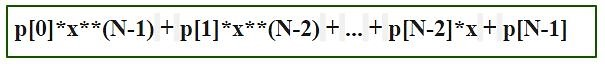# numpy.polyval() in Python

numpy.polyval(p, x) method evaluates a polynomial at specific values.

If ‘N’ is the length of polynomial ‘p’, then this function returns the value`Parameters : `
p : [array_like or poly1D] polynomial coefficients are given in decreasing order of powers. If the second parameter (root) is set to True then array values are the roots of the polynomial equation.
For example : poly1d(3, 2, 6) = 3x2 + 2x + 6

x : [array_like or poly1D] A number, an array of numbers, for evaluating ‘p’.

`Return:` Evaluated value of polynomial.

Code : Python code explaining polyval()

 `# Python code explaining  ` `# numpy.polyval() ` `   `  `# importing libraries ` `import` `numpy as np ` `import` `pandas as pd ` `   `  `# Constructing polynomial  ` `p1 ``=` `np.poly1d([``1``, ``2``])  ` `p2 ``=` `np.poly1d([``4``, ``9``, ``5``, ``4``])  ` `   `  `print` `(``"P1 : "``, p1)  ` `print` `(``"\n p2 : \n"``, p2)  ``# Solve for x = 2  ` `print` `(``"\n\np1 at x = 2 : "``, p1(``2``))  ` `print` `(``"p2 at x = 2 : "``, p2(``2``))  ``a ``=` `np.polyval([``1``, ``2``], ``2``) ` `b ``=` `np.polyval([``4``, ``9``, ``5``, ``4``], ``2``) ` ` `  `print` `(``"\n\nUsing polyval"``) ` `print` `(``"p1 at x = 2 : "``, a)  ` `print` `(``"p2 at x = 2 : "``, b)  ` ` `  `c ``=` `np.polyval(np.poly1d([``4``, ``9``, ``5``, ``4``]), np.poly1d(``2``)) ` `print` `(``"\nc : "``, c) `My Personal Notes arrow_drop_upIf you like GeeksforGeeks and would like to contribute, you can also write an article using contribute.geeksforgeeks.org or mail your article to contribute@geeksforgeeks.org. See your article appearing on the GeeksforGeeks main page and help other Geeks.

Please Improve this article if you find anything incorrect by clicking on the "Improve Article" button below.

Article Tags :

Be the First to upvote.

Please write to us at contribute@geeksforgeeks.org to report any issue with the above content.